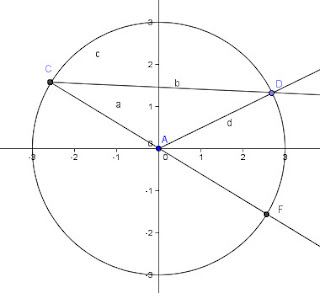## Thursday, 3 March 2011

### Ode to the Inscribed Angle

I was a little critical recently of Alex Bellos' treatment of the inscribed angle relation in his coverage of the Statue Problem in his book, "Here's Looking at Euclid".  After a little self-examination I realize that my response in calculus and pre-calc is to say something like, "Hey, you're supposed to know that... that's one of those simple little proofs you learned in geometry."  So in case you're one of my students and I've never shown you the "simple little proof", here is one using basic triangle properties.We begin by assuming that one side of the angle passes through the center of the circle and then render other cases as a variation of that case.  [For the purist, I realize that I have omitted the case where the inscribed angle is on the smaller arc that cuts the same segment which would force me to use arc-measures instead of angle measures]

Let DAF be the central angle that cuts the same arc as DCF, the inscribed angle.  Note that the distances AC and AD are both radii of the circle, and so CAD is an isosceles triangle with base angles ACD and ADC of equal measure.  So if we let x= the measure of ACD and ADC then CAD is 180-2x.  And since DAF forms a linear pair with CAD, it must be 2x... and thus it is shown.

If we had an inscribed angle that did not have one side passing through the center, then either the center is inside, or outside the angle.  We take each case individually.
We will let angle DCG be the inscribed angle, and DAG be the central angle. It is almost obvious after the last case that we can break DCG into two angles, DCA =x and ACG = y and using the same idea as the previous case we have DAG = 2x + 2y and again we are done.

The third case calls upon the use of angle subtraction and follows very easily from the first two.  Just move G up somewhere between F and D.  The rest, as the British say, is "easy-peasy"